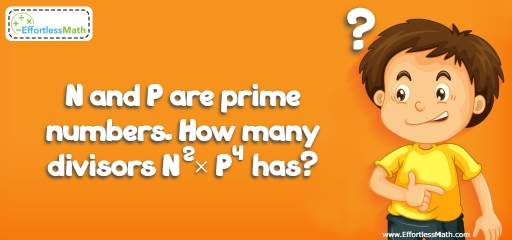# Geometry Puzzle – Critical Thinking 19

Critical thinking involves mindful communication. To develop your creative thinking, use this kind of math puzzle. The solution is also provided.## Challenge:

N and P are prime numbers. How many divisors N$$^2$$ × P$$^4$$ has?

### The Absolute Best Book to Challenge Your Smart Student!

If p and q are prime numbers, then for p$$^m$$q$$^n$$, the number of divisors is (m+1)(n+1).
Thus the number of divisors for N$$^2$$ × P$$^4$$ is (2+1)(4+1) = 15
Another way to solve this problem is by providing numbers for N and P. Let’s put 2 for N and 3 for P. Then, the value of N$$^2$$ × P$$^4$$ is 2$$^2$$ × 3$$^4$$ = 324
Now, find the factors of 324.
324: 1, 2, 3, 4, 6, 9, 12, 18, 27, 36, 54, 81, 108, 162, 324
324 have 15 factors.

The Absolute Best Books to Ace Algebra

### What people say about "Geometry Puzzle – Critical Thinking 19 - Effortless Math: We Help Students Learn to LOVE Mathematics"?

No one replied yet.

X
45% OFF

Limited time only!

Save Over 45%

SAVE $40 It was$89.99 now it is \$49.99